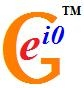# User blogs

Tag search results for: "other games"Nonlinear Theory of Elementary Particles Part XII: The Interaction Description (by Alexander G. Kyriakos): Abstract: In the present article it is shown, that all known interactions of elementary particles (except for gravity) have as a basis the electromagnetic interactions. In particular the classical (linear) electrodynamics describes only the 4-vector interaction. In the same time the nonlinear electrodynamics, or more general, the nonlinear theory of elementary particles (NTEP) includes both vector and axial interaction, integrating it into a unified electroweak interaction, and also the strong interaction. http://prespacetime.com/index.php/pst/article/view/226

Is the Master Formula for the U-matrix Finally Found? (by Matti Pitkänen): Abstract: In zero energy ontology U-matrix replaces S-matrix as the fundamental object characterizing the predictions of the theory. U-matrix is defined between zero energy states and its orthogonal rows define what I call M-matrices, which are analogous to thermal S-matrices of thermal QFTs. M-matrix defines the time-like entanglement coefficients between positive and negative energy parts of the zero energy state. M-matrices identifiable as hermitian square roots of density matrices. In this article it is shown that M-matrices form in a natural manner a generalization of Kac-Moody type algebra acting as symmetries of M-matrices and U-matrix and that the space of zero energy states has therefore Lie algebra structure so that quantum states act as their own symmetries. The generators of this algebra are multilocal with respect to partonic 2-surfaces just as Yangian algebras are multilocal with respect to points of Minkowski space and therefore define generalization of the Yangian algebra appearing in the Grassmannian twijstor approach to N = 4 SUSY. http://prespacetime.com/index.php/pst/article/view/227